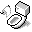Combinations

A binomial coefficient indicates how many different combinations of choices can be made from set of choices. This is very frequently used in figuring out probability, by determining first how many possible combinations exist.

## Equation

So, givendistinct choices, if we choosetimes, then the total number of different combinations we can get is:Ifis less than zero, or ifis greater than, thenequals to zero.

## Example

If there arered balls,green balls, andblue balls in a bag, and one randomly takes out two balls. What is the chance of getting two balls of the same color?

To answer this question, we first need to understand how many combinations/choices are available when drawing the balls from the bag. The total number of combinations from the two drawings is:equals. Out of those choices, we have 3 subsets of different colored balls. Drawing same colored ball is the same as drawing from the 3 subsets. Therefore the number of combinations for each color are:So the probability of two balls of the same color from a bag with 3 different colored balls is:## Other Notes

The equation and example above assumed that choices are not eliminated when chosen (without repetitions). If the same choice can be selected again, then the total number of combinations is:## References

1. Wikipedia contributors. Combinatorics. May 23, 2008 <http://en.wikipedia.org/wiki/Combinatorics>.
2. Wikipedia contributors. Binomial coefficient. May 23, 2008 <http://en.wikipedia.org/wiki/Binomial_coefficient>.Document is accessible from http://www.puchu.net. © 2002-2010 Sean Yang, Karen Yang, Don Yang and/or respective authors, all rights reserverd. This material may contain (biased) opinions, inappropriate materials for numerous individuals, work of other authors from the internet, links that refer to other web documents and resources, or origial work that cannot be use for personal or commercial purposes. Please respect the work of original authors.